Next:9.4 ShellsortUp:9. Sorting MethodsPrevious:9.2 Insertion Sort

# 9.3 Selection Sort

ith Iteration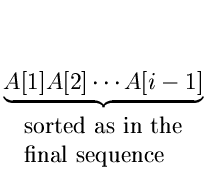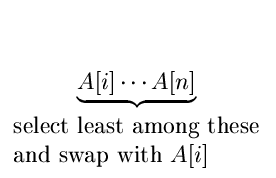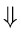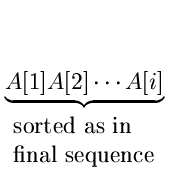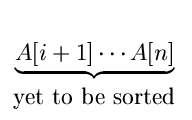{

for(i = 1, i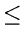n - 1;i + +)

{

lowindex = i; lowkey = A[i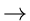key;

for(j = i + 1;jn;j + +)

if (A[jkey < lowkey)

{

lowkey = A[jkey;

lowindex = j

}

swap (A[i], A[lowindex])

}

}

• Number of swaps = n - 1
• Number of comparisons =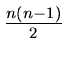eEL,CSA_Dept,IISc,Bangalore